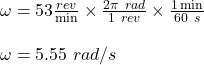## PLEASE HELP A ball, 1.8 kg, is attached to the end of a rope and spun in a horizontal circle above a student’s head. As the stud

Question

A ball, 1.8 kg, is attached to the end of a rope and spun in a horizontal circle above a student’s head. As the student rotates the ball in a horizontal clockwise circle their lab partner counts 53 rotations in one minute. What is the ball’s angular velocity in radians per second?

in progress 0
5 months 2021-08-27T07:00:45+00:00 1 Answers 3 views 0

The angular speed of the ball in radians per second is 5.55 rad/s.

Explanation:

Given;

mass of the ball, m = 1.8 kg

number of the ball’s rotation per minute, n = 53 RPM

The angular speed of the ball in radians per second is calculated as follows;Therefore, the angular speed of the ball in radians per second is 5.55 rad/s.